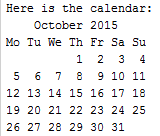# Python: Built-In Functions

#### Example: time library and sleep function (to pause programs)

In the example below, the sleep "function" within the time "library" (or package) is pausing program execution for two seconds. The import command brings in (imports) the specifed library, in this case the time library. Functions are synonymous with "methods".

``````
import time
print ("Thinking….")
time.sleep(2)
print("Done")
``````

Any Python file is a module. A package is a collection of Python modules. Both can be imported. One can also import downloaded/installed modules/packages, or create and import their own user-defined modules/packages.

#### Example: len and lower string methods

In this example, lower() is a function/method that returns the strong converted to lower case. len() is a built-in function that returns the numeric length of a string.

``````
name1 = "DAN SMITH"
print(name1.lower(), "\n")
name2 = input('Enter your name: ').lower()
print (name2, "\n\n\n")
print ("Your name is", len(name2), "characters long")
``````

Output:

dan smith
Enter your name: BOB JONES {user input}
bob jones
Your name is 9 characters long

#### Example: Replace function

The replace() function (method) returns a copy of a string that hsa had some of the text replaced with different text.

``````
text = "The quick brown fox jumps over the lazy dog."
text = text.replace("fox", "dog")
text = text.replace("lazy dog", "lazy fox")
print (text)
``````

Output:

The quick brown dog jumps over the lazy fox.

#### Example: calender library and month function

``````
import calendar
cal = calendar.month(2015, 10)
print ("Here is the calendar:")
print (cal)
``````

Output:#### Example: A few math functions

``````
import math

total1 = math.pow(5,2)
total2 = math.sqrt(36)
total3 = math.pi * 2.5

print ("Total1 = ", total1)
print ("Total2 = ", total2)
print ("Total3 = ", total3)
``````

Output:

Total1 = 25.0
Total2 = 6.0
Total3 = 7.853981633974483

#### Example: Random function

``````
import random

die1 = random.randrange(1,7)
die2 = random.randrange(1,7)
print ("Dice 1 roll: ", die1)
print ("Dice 2 roll: ", die2)

card_deck = ['2C', '2H', '2S', '2D', '3C', '3H', '3S', '3D']
random.shuffle(card_deck)
print ("Top card on deck: ", card_deck)
``````

Output:

Dice 1 roll: 3
Dice 2 roll: 6
Top card on deck: 2S

Dice 1 roll: 2
Dice 2 roll: 5
Top card on deck: 2D

#### Example: Miscellaneous Built-In Functions

``````
import math, random, datetime, os, calendar, time

name = input("Please enter your full name: ")
num1 = float(input("Please enter number for calculations: "))

areaC = math.pi * math.pow(num1,2)
squareRoot = math.sqrt(num1)
ran1 = random.randrange(1,num1)
cal = calendar.month(2017, 1)

os.system('cls')
print("The area of the circle is: %.2f" % areaC + ".")
print("Square root of number is: ", str(squareRoot) +  ".")
print("Random number between 1 and", int(num1), "is: ", str(ran1) + ".")
print ("Current Date: " ,datetime.datetime.now().strftime("%m/%d/%y."))
print ("Your name is", len(name), "characters long.")
print (cal)
print ("Thinking….")
time.sleep(2)
print("Done")

card_deck = ['2C', '2H', '2S', '2D', '3C', '3H', '3S', '3D']
random.shuffle(card_deck)
print ("Top card on deck: ", card_deck)

os.startfile("diagnose_power.pdf")
``````

Output:

Please enter your full name: John Smith
Please enter number for calculations: 25

The area of the circle is: 1963.50.
Square root of number is: 5.0.
Random number between 1 and 25 is: 20.
Current Date: 01/21/17.
Your name is 10 characters long.
January 2017
Mo Tu We Th Fr Sa Su
1
2   3    4    5    6   7    8
9 10 11 12 13 14 15
16 17 18 19 20 21 22
23 24 25 26 27 28 29
30 31

Thinking….
Done
Top card on deck: 3C

#### Example: A few operating systems functions

``````
import os
os.system('cls')       # clear screen in Windows
for filename in os.listdir("C:\\"):  # display directory
print (filename)
``````

#### Example: Running a function in an external Python file

This program runs the diagnostic_menu() function in the diagnose.py external file located in the same folder/directory

``````
import diagnose
``````

#### Example: Use of import function to call/use external Python files (user-defined libraries)

filename: program1.py

``````
import my_library

# Main
num = int(input("Please enter max number: "))
my_library.displayText(num)
``````

filename: my_library.py

``````
def displayText(num):
for x in range(num):
print("Count: ", x)
``````

Output (from running program1.py):

Please enter max number: 3 {user input}
Count: 0
Count: 1
Count: 2

#### Example: while loop menu using same external Python file

filename: program2.py

``````
import my_library

main_choice=True
while main_choice:
print("    Main Menu    ")
print("------------------")
print("1. Counter Program")
print("2. Exit")
main_choice = input("Please enter menu choice (1-2): ")
if main_choice == "1":
num = int(input("Please enter max number: "))
my_library.displayText(num)
elif main_choice == "2":
print("Goodbye.")
main_choice=False
else:
``````

#### Example: Built-in functions to call/open an external file

``````
import os
os.startfile("diagnose_power.pdf")
import webbrowser
webbrowser.open_new(r'diagnose_power.pdf')
import subprocess
subprocess.Popen("diagnose_power.pdf",shell=True)
# Mac syntax: os.system('open diagnose_power.pdf')
``````

#### Example: Data In and Data Out Function and Function Call from Print Statement

``````
import math
area = math.pi * (radius ** radius)
return area

print("Calculate the area of a circle")
print("The area of the circle is %.2f" % area)

print("The area of the circle is %.2f" %  calcCirc(radius))
``````

Output:

Calculate the area of a circle
The area of the circle is 84.82
The area of the circle is 84.82

#### Example: Join function

``````
list1 = ["The", "quick", "fox", "runs."]
sent = " ".join(list1)
print (sent)
``````

Output:

The quick fox runs.